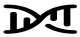# Horizontal Pleiotropy

A key way in which MR may violate the exclusion restriction assumption. Also known as “genuine” or “true” pleiotropy. This is when a genetic variant affects other traits which influence the outcome independently of the hypothesised exposure.

This can result in biased MR estimates because of violation of the exclusion restriction assumption. For example, if some or all of the genetic IVs that robustly associate with the risk factor of interest also, independently of the association with the risk factor, associate with other risk factors for the outcome, then the MR estimate will be the combined effect of all of these (independent) risk factors – not the effect of the risk factor of interest alone. Methods such as MR-Egger, weighted median- and mode-based MR methods have been developed to explore and account for the impact of horizontal pleiotropy in MR studies. This can also be informative about the trait’s aetiology (see MR-TRYX).Figure 2.4 - One-sample Mendelian randomization. (A) MR relies on the following three core assumptions: (1) the genetic variant(s) being used as an instrument (Z) is associated with the exposure (X); (2) the instrument in independent of measured and unmeasured confounders (U) of the association between the exposure (X) and outcome (Y); and (3) there is no independent pathway between the instrument (Z) and outcome (Y) other than through the exposure (X) – otherwise known as horizontal pleiotropy or the exclusion restriction assumption. (B) MR can be perceived as being analogous to a randomized controlled trial (RCT), whereby the random assortment of alleles at conception is equivalent to the randomization method with an RCT. This randomization process produces groups of individuals who differ with respect to the intervention (in the case of MR, genetic variation) and between which confounders are equally distributed. Therefore, any differences observed in the outcome of interest between these randomly allocated groups should be due to the exposure with which the genetic variant(s) are associated. (C) In the most simple of scenarios, the causal estimate of the association between the exposure (X) and outcome (Y) can be derived using the instrumental variable ratio method (otherwise known as the Wald ratio), where 𝛽𝐼𝑉 is the causal estimate derived from instrumental variable (i.e., MR) analyses, 𝛽𝑌𝑍 is the association between the instrument (Z) and the outcome (Y) and 𝛽𝑋𝑍 is the association between the instrument (Z) and the exposure (X).Figure 4.2 - Vertical and Horizontal Pleiotropy. Adapted from Hemani et al. and Holmes et al. (A) Classic horizontal pleiotropy, whereby the instrument (Z) for the exposure of interest (X) is independently associated with the outcome (Y) either directly or indirectly through other trait(s) – denoted “?”. Here, this would violate the third assumption of MR and would bias results from an MR study. (B) Indirect horizontal pleiotropy, whereby another SNP (Z2) in linkage disequilibrium (LD) with the instrument (Z1) for the exposure of interest (X) is associated with the outcome (Y) and, due to this correlation between SNPs, the instrument is therefore not independent of the outcome of interest. This is another reason to use independent genetic variants as instruments in an MR analysis and to have some biological knowledge about the mechanisms by which the SNPs are associated with the exposure. (C) A depiction of vertical pleiotropy, whereby the genetic instrument (Z) for the exposure (X) is associated with other trait(s) – denoted “?” – however, this reflects the downstream effects of the exposure that is likely on the causal pathway linking the exposure to the outcome (Y). This is the very essence of MR and is not something that needs to be accounted for in analyses. Measured and unmeasured confounders in all diagrams as represented by “U”, “U1” and “U2”.